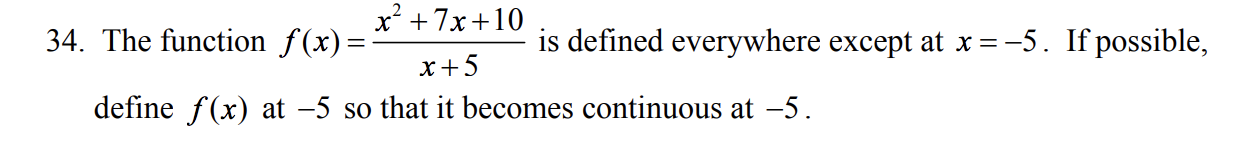# x2 +7x +10 x+5 define f(x) at -5 so that it becomes continuous at -5 34. The function f(x)- is defined everywhere except at x--5. If possible,

Question

sketch the graph and find the x-values (if any) at which f is not
continuous. Classify the discontinuities as being removable or essential. If the latter, state
whether it is a jump discontinuity, an infinite discontinuity, or neither.help_outlineImage Transcriptionclosex2 +7x +10 x+5 define f(x) at -5 so that it becomes continuous at -5 34. The function f(x)- is defined everywhere except at x--5. If possible, fullscreen

### Want to see the step-by-step answer?